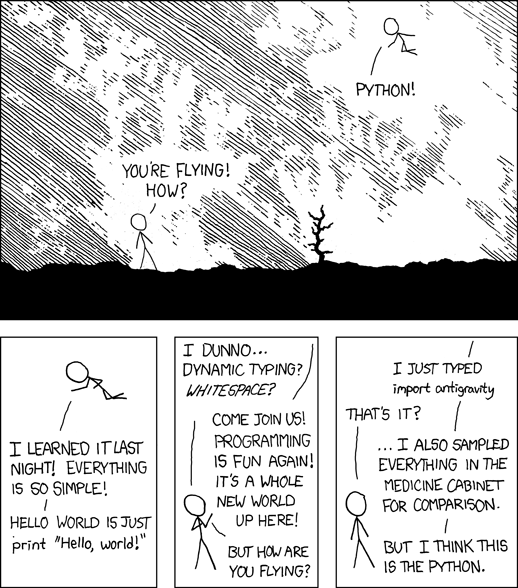Eric's Programming Page

General information

The author of the this webpage is Eric Reichwein. He got interesting in programming while studying physics at the University of California, Santa Cruz. His interests are mainly in experimental condensed matter physics and photonics, which require a lot of data analysis computations. Below is small showcase of some of the cool physics programming and simulations Eric has done.

Eric's Coding Projects

The code will be posted ASAP. I want to embed the code in a nice display, which I am working on now (slowly).Eric's Programs

Band Structure Calculation

Results

TIGHT BINDING MODEL ON SQUARE LATTICETIGHT BINDING MODEL FOR GRAPHENEProgram Description

We calculated the 2D band structure using the Tight Binding Model. We first calculated the band structure of a square lattice. Then we attacked the graphene (hexagonal lattice) band structure. Along with both of these (square lattice and hexagonal) we calculated the density of states by making a histogram of energies. We used the tight binding model for these calculations. It takes approximately 20 lines of SciPy code for these results.

2D Band Structure Calculation movie through energy bands

Program Description

Using an extension of the Wigner-Seitz method (Kittel: Chapter 9, equation 17). Northeastern has a nice description of this method. Basically, the tight binding model is very limited. The main thing is discretization of the Schroedinger equation. The video shows different energy levels. Red being higher energy and blue lower energy.

1D Ising Model

Program Description

1D Ising model of a spin system. The black corresponds to spin down and white is spin up. It is a little bland, but I assure it is very, very cool. The 2D spin is more visually aesthetic. This is a Monte Carlo simulation. We look at the energy of the spin system then chose random probabilities if it would flip (which was based on temperature).

2D Ising Model

Monte Carlo Ising model in 2D.

Results

ISING MODEL WHEN ABOVE CRITICAL TEMPERATURE

Above the critical temperature we see random evenly distributed magnetization (or number of spins aligned).

ISING MODEL WHEN AT THE CRITICAL TEMPERATURE

At the critical temperature we get bizarre behavior. This is due to what we call a phase transition. This is when there is non-analytical behavior of properties of the system. Here we see the polarization of the spins acting sporadic. It goes from most up to most down to most up etc. This is due to the strange behavior of phase transitions.

ISING MODEL WHEN BELOW CRITICAL TEMPERATURE

We see that the polarization of the spins (or magnetization) pretty quickly go to one direction. That video is not that interesting (just goes white) so here is one just barely below the critical temperature so we some typical phase transition behavior.

Program Description

2D Ising model of a spin system. The black corresponds to spin down and white is spin up. It is the same idea as the 1D model except now the Hamiltonian (energy of the nearest neighbors interaction) has two indices instead.

XY Model

Monte Carlo XY model in 2D.

Results

XY MODEL HIGH TEMPERATURE

We used quiver to display the arrows. The arrows represent the electron spin. The interaction energy now has to have cosine which slows down the computation. We display the power spectrum as well using fourier transforms and inverse fourier transforms.

XY MODEL LOW TEMPERATURE

The same model just with setting beta high (beta is inversely proportional to temperature).

Time Evolution of the tight binding model

Program Description

This is a video of the time evolution of the tight binding model. It is very similar to diffusion. The red is higher value of wavefunction, blue is lower (wavefunction squared is probability). We know (as stated above) that the tight binding model is too crude to produce accurate results, but it does produce a nice video.

Blackjack Simulator and Card Counter

Program Description

This was one of my first Python programs I made. I have been interested in probability (especially in gaming and gambling) for a while. So it was a natural instinct to make a program that will help me become rain man. I would like to think that it helped me win \$67 in 3 hours at a \$2 blackjack table in Reno. It may no be the prettiest widget, but it does work. If you don't believe me try it out. Here is the text file of the python script. Looking at the code you will need to know how tkinter works. You can find a Link to tkInter API